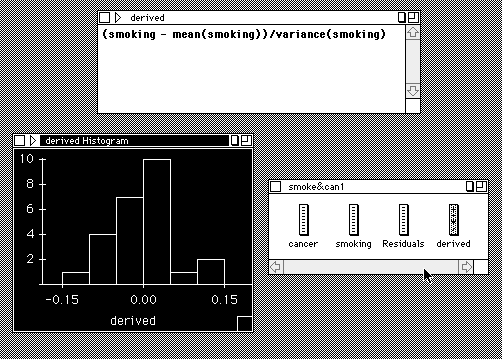Derived Variables

These are either created from the Transform menu of Calc or from New of the Data menu.

Although menu based choices of selected icons may be used, derived variables are recorded textually. Doubleclicking on a derived variable created by a menu command will illustrate how the language of derived variables works.

Arithmetic
expressions like '(5*X[i] - 3*Y[i])/Mean(X)' are allowed.
If ... Then ... Else ...
is possible and illustrated in the string example below.
Sample Derived VariableString
data can be generated using double quotiation marks, e.g.
```		if assets > 10000 then
"Big"
else if assets > 2000 then
"Medium"
else
"Small"
```
A string Variable_Name
(possibly within backquotes) may be used in a derived variable to refer to another. The value of this expression for each case will be the value of that case in the variable named by Variable_Name.
'Variable_Name[i]'
refers to the i'th case of the variable named by Variable_Name.